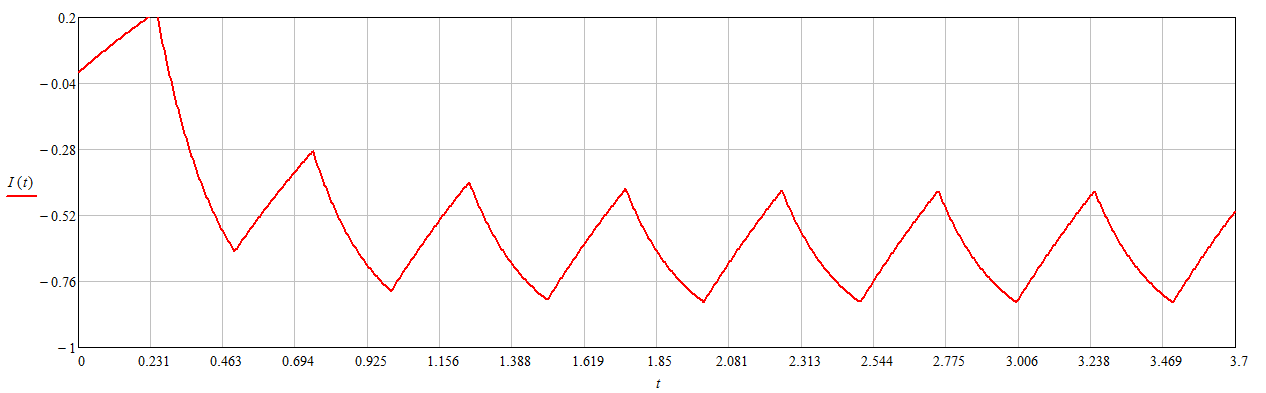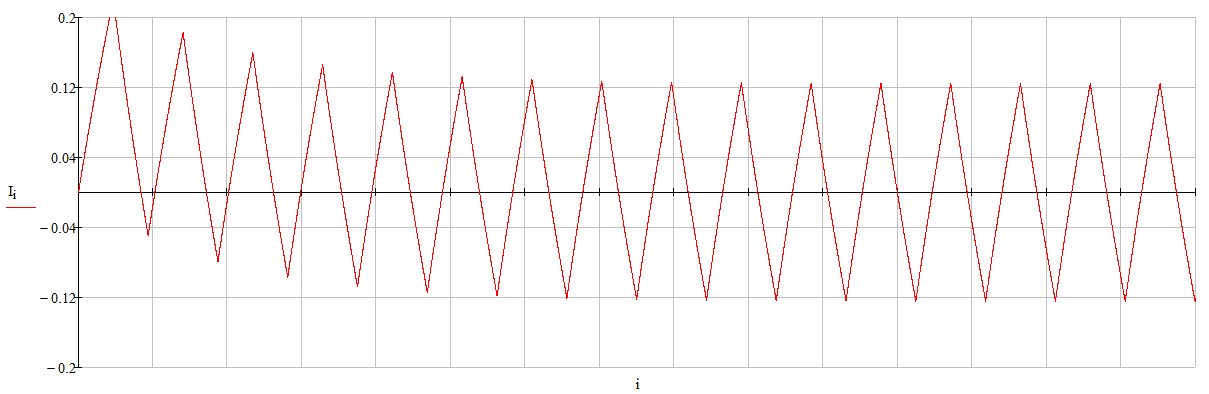Research website of Vyacheslav Gorchilin
2021-08-01
Parametric generators of the second kind
Parametric generation of electrical energy has been known for a long time and is well covered in works [1-5]. The generation methods proposed there are based on a parametric change in capacitance or inductance, which are included in an electrical circuit without an additional power source. But just such a source can turn such a device into a full-fledged generator of free energy. The condition for this is the operation of the generator in a parametric circuit of the second kind.
This note will show the fundamental possibility and some approaches to a multiple increase Efficiency of the second kind on specific examples of parametric generators of the second kind (PMG), moreover, all this will be done exclusively by classical methods adopted in electrical engineering and electrodynamics for calculating electrical circuits, without going beyond the classical concepts of the physics of processes. The author hopes that this note will allow researchers to take a fresh look at the problem of parametric generators and make their efforts more productive.
This work opens a cycle of notes on parametric circuits of the second kind, the difference from the chains of the first kind can be found here and here. On this basis, and by analogy, we will consider the SGP, the main feature of which is the independence of the master and parametric systems (Fig. 1a). The driving system (G1) is responsible for generating electrical power to transfer it to the load Rn through the parametric element PME1, and the second (G2) is responsible for changing the parameter of this element. The approach presented here will allow this principle to be extended not only to the number of electrical, but also to the electromechanical machines.
In more detail, we work here a special case of such generators - a circuit containing an inductance L, which here represents a parametric element, whose parameter changes depending on the characteristics of G2 (Fig. 1b). The voltage source G1 generates oscillations, the shape of which can be, in principle, any: from sinusoidal to pulsed. This voltage is applied to the series-connected inductance L and resistance R, which together create a current I flowing through this circuit. The inductance of the coil L changes according to the known law, which generates G2. It is on this scheme that we will rely in the subsequent presentation.Fig.1. Block diagram of the second type PMG (a), schematic diagram of the second type PMG (b)
In this paper, we will mean the ideal case when G1 does not affect G2 in any way, and vice versa. In reality, you will need to strive for this. For example, one can partially approach ideal conditions if a transformer with orgotonal windings is used as a coil L, where one of them is connected to G1 and will be through, and the other will change the magnetic permeability of the core of this transformer depending on G2. A similar transformer is suggested, for example, here.
From the circuit in Figure 1b, we can immediately write the equation of the electrical circuit, according to Kirchhoff's law: $L (t) \, \dot I + R I = U (t) \qquad (1.1)$ Here: $$I$$ is the current in the circuit, $$\dot I = dI / dt$$ is the time derivative of the current, $$R$$ is the load resistance, $$U (t)$$ - vibration voltage G1, which can have different shapes. The inductance $$L$$ changes from the parameter given by G2, but synchronized in time with G1. In this case: $$L = L (t)$$.
In fact, in equation (1.1) there should be one more term: $$I\, dL / dt$$, but since we will consider idealized pulses with zero time rise and fall, then this term turns out to be very small and we equate it to zero.
Important. In this work, we do not take into account the energy that is spent on changing the inductance parameter. In reality, everything will depend on the method, or even the physical principle, that changes this parameter. The total balance of the received capacities in a real device may turn out to be either more or less than the expended ones.
Let's get down to business right away and experiment with rectangular pulses, and at the same time select a methodology for such studies. For example, suppose generator G1 generates square-wave pulses with values "1" and "-1" (Fig. 2a). In the same time intervals, the generator G2 changes the inductance parameter from "1" to "0.2", that is, the inductance changes five times (Fig. 2b). Thus, in the task at hand, we must answer two questions: how does the current through the load change and what power balance we will get as a result.Fig.2. Graph of pulses of the generator G1 (a), change in inductance L (b and c)
To answer the questions posed, you can solve the differential equation (1.1) in a special mathematical editor, but, as it turned out, for some values of the circuit elements, the algorithms of such editors fail and produce incorrect results. To solve such an equation analytically is either difficult or impossible at all. Therefore, we will go the other way and take as a basis the stable step-by-step calculation algorithm presented by here.
Formula (1.1) is the Bernoulli equation, which, by the way, contains the key to the search for free energy. Let's not clutter the search for this formula with calculations and immediately write down its step-by-step solution: $I_i = {L_i \, I_ {i-1} + U_i \, \Delta t \over L_i + R \, \Delta t} \qquad (1.2)$ Where: $$i$$ is the number of the iteration step, which starts with one and ends with $$N$$, which determines the number of oscillations multiplied by the number of points to construct one oscillation. In this case, we assume that the initial value of the current is equal to zero: $$I_0 = 0$$. We take the iteration step equal to 1/4, because this is exactly how many points contain one oscillation according to Figure 2, then: $$\Delta t = 0.25$$. Also, for a start, let's take the value of the load resistance equal to one: $$R = 1$$.
You can find the current values according to formula (1.2) step by step manually, but it is easier for a computer to do it. Let's take 16 points for plotting ($$N = 16$$) and build a graph using MathCAD (Fig. 3). You can check the accuracy of the construction if you take the standard MathCAD solution to diff. equations and 50,000 points. Then the graph will be converted to Figure 4.Fig.3. Current graph built from 16 points using an iterative algorithmFig.4. Current graph, built from 50,000 points according to the internal algorithm of the math editor
Although the 16-point graph in Figure 3 is not as accurate as in Figure 4, consisting of 50,000 points, it also shows the main difference between the second type PMG from the classic one: the graph slides into the negative area of the current, which provides an increase in efficiency . Immediately, you need to add the fact that the current graph can be shifted to the positive region, but for this you need to rotate the change in inductance relative to the voltage by 180 degrees, as in Figure 2c. It is interesting that in these cases the inductance L acquires the properties of negative resistance  and becomes an additional source of energy in the parametric circuit. But this shift works only in cases where the frequencies G1 and G2 coincide, which in itself is already interesting, since according to the classics, the parametric starts to work at the doubled frequency in G2 relative to G1.Fig.5. Non-parametric circuit current graph
For comparison, just above is a graph of the current in the circuit, where the parametric change in inductance does not occur and $$L = 1$$ always. It is clearly seen that in this case, after a steady state, the oscillations become symmetric about zero (Fig. 5), and their integral sum tends to zero. There is no increase in efficiency here.
Power balance
Since we now have a reliable tool for finding solutions to equation (1.1) and an editor, the number of iteration points can be increased, which we will do in subsequent calculations. In the meantime, we need to answer the second most important question of the task and find a balance of capacities for our SGP. To do this, we first obtain the power dissipation in the active load R: $P_R = {R \over N} \sum_ {i = 1} ^ N (I_i) ^ 2 \qquad (1.3)$ Here: $$N$$ is the number of points for plotting the graph. For example, for Figure 3, the number of points is four: $$N = 16$$. The more such points there are, the more accurate the result will be.
Now let's find the power consumed by the power supply: $P_U = {1 \over N} \sum_ {i = 1} ^ N (U_i \, I_i) \qquad (1.4)$ It should be recalled that in this work we do not consider the power of the generator G2 (Fig. 1a), spent on changing the inductance parameter, but in the balance of capacities, we must take it into account: $K _ {\eta 2} = {P_R \over P_U + P_ {pm}} \qquad (1.5)$ Here: $$K _ {\eta 2}$$ - growth efficiency of the second kind, from which the coefficient immediately follows COP efficiency factor; $$P_ {pm}$$ - power consumed to change the inductance parameter, which we equate to zero for now.
Based on these formulas, in the second part, we will analyze examples of parametric generators with different pulse shapes and frequency ratios, Let us draw some conclusions about the sources of energy increments in such systems.

1 2Materials used
1. Mandelstamm L.I., Papaleksi N.D. On the excitation of oscillations in an electric oscillatory system by means of a periodic change in capacitance. [PDF]
2. Mandelstamm L.I., Papaleksi N.D. On parametric excitation of electrical oscillations. [PDF]
3. Lazarev V.A. On heteroparametric excitation. [PDF]
4. Gulyaev V.I., Migulin V.V. On the stability of oscillatory systems with periodically changing parameters. [PDF]
5. Zatsarinin S.B. Parametric generation of electrical energy. [PDF]
6. Karasev M.D. Some general properties of nonlinear reactive elements. UFN 69 217-267 (1959)